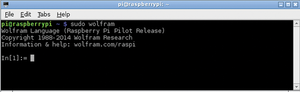# Mathematica and the Wolfram language on Raspberry Pi

#### Getting Started

With the Wolfram Engine on the Raspberry Pi, you effectively have two ways of programming with the Wolfram language. The command-line version of the Wolfram language lets you execute commands from an LXTerminal command-line session or from a previously saved executable `.m` script. The alternative method is to use the Mathematica notebook interface, which is a word-processor-like environment with the added capability of really intuitive programming and interaction with the Wolfram language. This approach is similar to programming in other languages using an Integrated Development Environment (IDE). However, the word processor interface of Mathematica adds a whole new level of capability that is not present in a conventional IDE: You can actually calculate in real time within a notebook, effectively adding programming capability to a word processing environment.

If you want to run the command-line program (which is not necessarily the best place to start for a beginner), either click on the Wolfram icon (the one that looks like a wolf) in the app launcher menu or double-click the desktop icon. Alternatively, you can open an LXTerminal session, type

`sudo wolfram`

and hit Enter; you should see an interface in the LXTerminal window that looks like Figure 4.Figure 4: Starting up Wolfram in a terminal window.

If you would prefer to work from the Mathematica user interface (which I recommend for the exercises in this article), simply launch Mathematica from the app launcher or double-click the desktop icon. You should then see a splash screen with the Mathematica "Spikey" logo (technically speaking, the shape is a hyberbolic dodecahedron with what Wolfram call "embelishments") hovering over the standard Raspberry Pi logo while the program loads (Figure  5). Once the program fully loads, two screens appear  – a blank notebook file and a help window (Figure 6).Figure 5: The Mathematica logo "Spikey" overs over the Raspberry background while the program loads.

If at any point you need or want to open a new notebook, click File | New | Notebook, or alternatively, you can just use the keyboard shortcut Ctrl+N. You can also save your work in a notebook by using the keyboard shortcut Ctrl+S or by clicking on File | Save.

In this article, I focus on the Mathematica user interface; however, all the commands should work from the command-line Wolfram interface as well. Additionally, it is important to note that I focus on the actual Wolfram language programming aspect of the Mathematica interface, rather than the word processing and layout features also available in the Mathematica notebook interface.

Customarily every programming lesson begins by outputing the string Hello world! In Mathematica, you just have to type:

`Print ["Hello world!"]`

In the Mathematica GUI, you then press Shift+Enter to execute the command, whereas if you are on the command line, you just press Enter. The result should be:

`Hello world!`

Although this simple program is somewhat gimmicky, it does introduce three important aspects of the Wolfram language:

• Functions
• Arguments
• Strings

I'll explain these concepts in the following sections, along with other important Wolfram components, such as lists and variables.

#### Functions

In the most basic terms, a function takes in an input, performs some kind of processing operation on it, and gives an output. For example, squaring or dividing a number is a function. The Wolfram language has more than 5,000 built-in functions, and it is even possible to create your own. In the Wolfram language, functions always start with a capital letter. In the preceding example, the function is `Print`.

Another example is the `N` function. Mathematica likes to keep numbers in the most exact form. For instance, when dividing in Mathematica, the software attempts either to divide the number perfectly into an integer value (i.e., 200/4 = 50) or, if the result is not an integer, to keep the answer in fraction notation, converting it to the simplest possible form (i.e., 200/6 = 100/3). However, sometimes you will want to know what the numerical version of a fraction is, and this is where the `N` function comes in. If you type

`1/3`

and press Shift+Enter, you get the output:

However, if you use the `N` function, type the code

`N[1/3]`

and press Shift+Enter, you get the output:

Express-Checkout as PDF

Pages: 2

Price \$2.95
(incl. VAT)